# axis

Set axis limits and aspect ratios

## Syntax

``axis(limits)``
``axis style``
``axis mode``
``axis ydirection``
``axis visibility``
``lim = axis``
``````[m,v,d] = axis('state')``````
``___ = axis(ax,___)``

## Description

example

````axis(limits)` specifies the limits for the current axes. Specify the limits as vector of four, six, or eight elements.```

example

````axis style` uses a predefined style to set the limits and scaling. For example, specify the style as `equal` to use equal data unit lengths along each axis.```

example

````axis mode` sets whether MATLAB® automatically chooses the limits or not. Specify the mode as `manual`, `auto`, or one of the semiautomatic options, such as `'auto x'`.```

example

````axis ydirection`, where `ydirection` is `ij`, places the origin at the upper left corner of the axes. The y values increase from top to bottom. The default for `ydirection` is `xy`, which places the origin at the lower left corner. The y values increase from bottom to top.```

example

````axis visibility`, where `visibility` is `off`, turns off the display of the axes background. Plots in the axes still display. The default for `visibility` is `on`, which displays the axes background.```

example

````lim = axis` returns the x-axis and y-axis limits for the current axes. For 3-D axes, it also returns the z-axis limits. For polar axes, it returns the theta-axis and r-axis limits.```
``````[m,v,d] = axis('state')``` returns the current settings for the axis limit selection, the axes visibility, and the y-axis direction. This syntax will be removed in a future release. Use the `XLimMode`, `YLimMode`, `ZLimMode`, `Visible`, and `YDir` properties of the axes to get the values instead.```

example

````___ = axis(ax,___)` uses the axes or polar axes specified by `ax` instead of the current axes. Specify `ax` as the first input argument for any of the previous syntaxes. Use single quotes around input arguments that are character vectors, such as `axis(ax,'equal')`.```

## Examples

collapse all

Plot the sine function.

```x = linspace(0,2*pi); y = sin(x); plot(x,y,'-o')```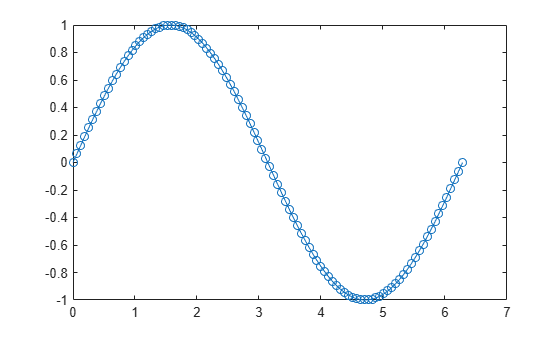Change the axis limits so that the x-axis ranges from $0$ to $2\pi$ and the y-axis ranges from -1.5 to 1.5.

`axis([0 2*pi -1.5 1.5])`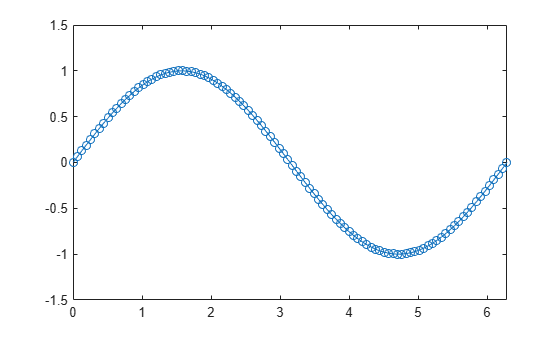Create a stairstep plot, and use the `axis padded` command to add a margin of padding between the plot and the plot box.

```x = 0:12; y = sin(x); stairs(x,y) axis padded```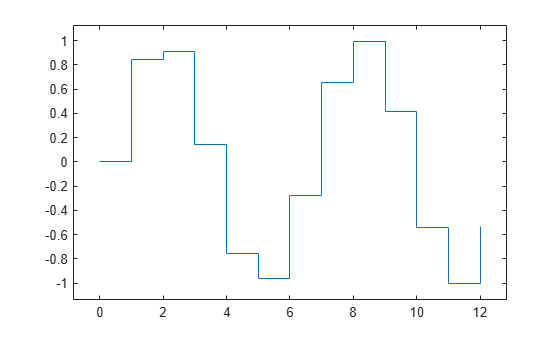Create a plot. Set the limits for the x-axis and set the minimum y-axis limit. Use an automatically calculated value for the maximum y-axis limit.

```x = linspace(-10,10,200); y = sin(4*x)./exp(.1*x); plot(x,y) axis([-10 10 0 inf])```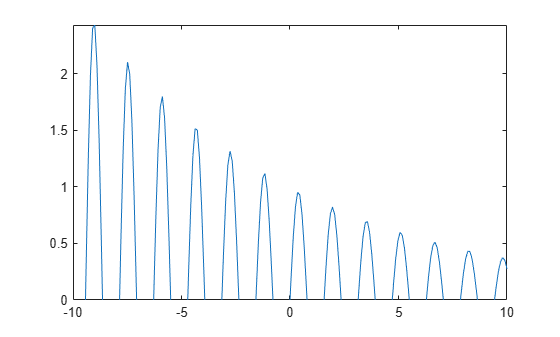Starting in R2019b, you can display a tiling of plots using the `tiledlayout` and `nexttile` functions. Call the `tiledlayout` function to create a 2-by-1 tiled chart layout. Call the `nexttile` function to create the axes objects `ax1` and `ax2`. Plot data in each axes. Then set the axis limits for both axes to the same values.

```tiledlayout(2,1) x1 = linspace(0,10,100); y1 = sin(x1); ax1 = nexttile; plot(ax1,x1,y1) x2 = linspace(0,5,100); y2 = sin(x2); ax2 = nexttile; plot(ax2,x2,y2) axis([ax1 ax2],[0 10 -1 1])```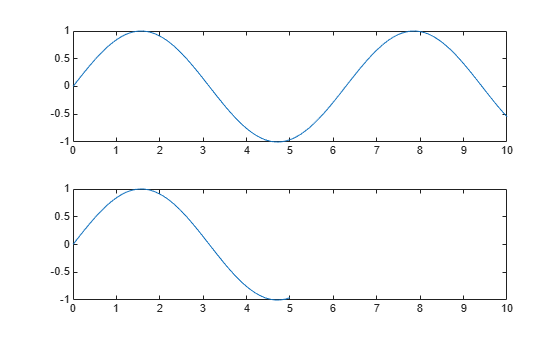Plot a surface without displaying the axes lines and background.

```surf(peaks) axis off```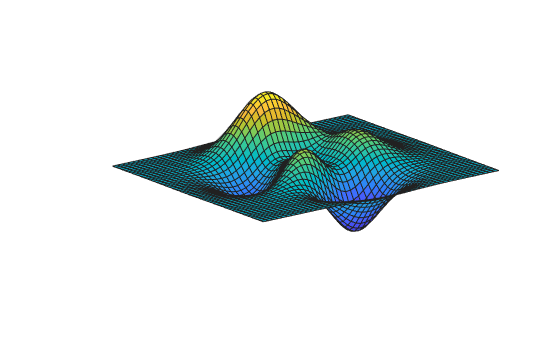Plot a surface. Set the axis limits to equal the range of the data so that the plot extends to the edges of the axes.

```surf(peaks) axis tight```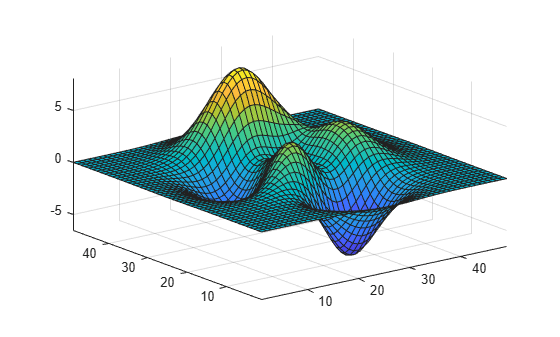Return the values of the current axis limits.

`l = axis`
```l = 1×6 1.0000 49.0000 1.0000 49.0000 -6.5466 8.0752 ```

Create a checkerboard plot and change the direction of the coordinate system.

First, create the plot using the `summer` colormap. By default, the x values increase from left to right and the y values increase from bottom to top.

```C = eye(10); pcolor(C) colormap summer```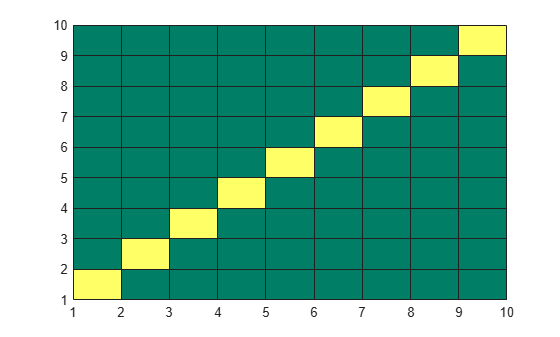Reverse the coordinate system so that the y values increase from top to bottom.

`axis ij`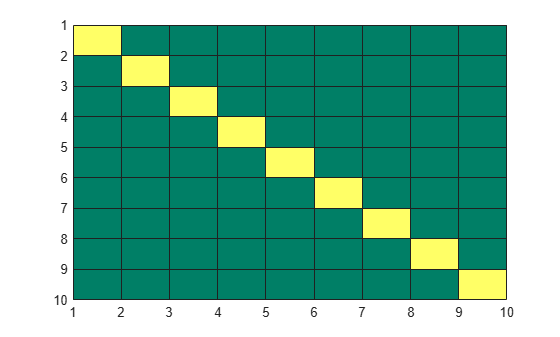Plot a sine wave.

```x = linspace(0,10); y = sin(x); plot(x,y)```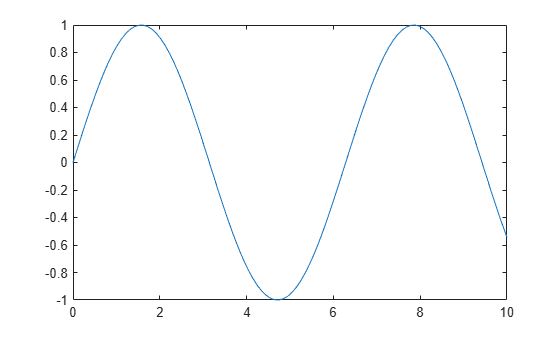Add another sine wave to the axes using `hold on`. Keep the current axis limits by setting the limits mode to manual.

```y2 = 2*sin(x); hold on axis manual plot(x,y2) hold off```If you want the axes to choose the appropriate limits, set the limits mode back to automatic.

`axis auto`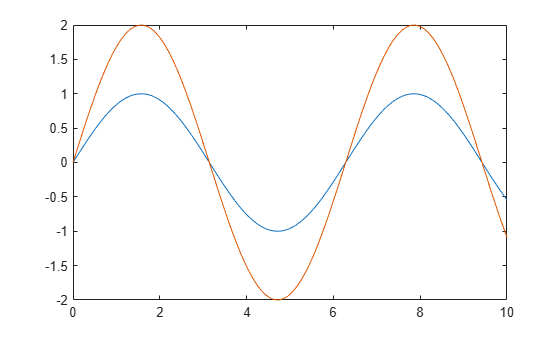## Input Arguments

collapse all

Axis limits, specified as a vector of four, six, or eight elements.

For Cartesian axes, specify the limits in one of these forms:

• `[xmin xmax ymin ymax]` — Set the x-axis limits to range from `xmin` to `xmax`. Set the y-axis limits to range from `ymin` to `ymax`.

• `[xmin xmax ymin ymax zmin zmax]` — Also set the z-axis limits to range from `zmin` to `zmax`.

• `[xmin xmax ymin ymax zmin zmax cmin cmax]` — Also set the color limits.` cmin` is the data value that corresponds to the first color in the colormap. `cmax` is the data value that corresponds to the last color in the colormap.

The `XLim`, `YLim`, `ZLim`, and `CLim` properties for the `Axes` object store the limit values.

For polar axes, specify the limits in this form:

• `[thetamin thetamax rmin rmax]` — Set the theta-axis limits to range from `thetamin` to `thetamax`. Set the r-axis limits to range from `rmin` to `rmax`.

The `ThetaLim` and `RLim` properties for the `PolarAxes` object store the limit values.

For partially automatic limits, use `inf` or `-inf` for the limits you want the axes to choose automatically. For example, ```axis([-inf 10 0 inf])``` lets the axes choose the appropriate minimum x-axis limit and maximum y-axis limit. It uses the specified values for the maximum x-axis limit and minimum y-axis limit.

Note

If the x-axis, y-axis, or z-axis displays categorical, datetime, or duration values, then use the `xlim`, `ylim`, and `zlim` functions to set the limits instead.

Example: `axis([0 1 0 1])`

Example: `axis([0 1 0 1 0 1])`

Example: `axis([0 inf 0 inf])`

Manual, automatic, or semiautomatic selection of axis limits, specified as one of the values in this table. All of the auto mode values use the `tickaligned` `style` to calculate the limits for the particular axis or set of axes you specify.

ValueDescriptionAxes Properties That Change
`manual` Freeze all axis limits at their current values. Sets `XLimMode`, `YLimMode`, and `ZLimMode` to `'manual'`. If you are working with polar axes, then this option sets `ThetaLimMode` and `RLimMode` to `'manual'`.
`auto`Automatically choose all axis limits. Sets `XLimMode`, `YLimMode`, and `ZLimMode` to `'auto'`. If you are working with polar axes, then this option sets `ThetaLimMode` and `RLimMode` to `'auto'`.
`'auto x'`Automatically choose the x-axis limits. Sets `XLimMode` to `'auto'`.
`'auto y'`Automatically choose the y-axis limits. Sets `YLimMode` to `'auto'`.
`'auto z'`Automatically choose the z-axis limits. Sets `ZLimMode` to `'auto'`.
`'auto xy'`Automatically choose the x-axis and y-axis limits. Sets `XLimMode` and `YLimMode` to `'auto'`.
`'auto xz'`Automatically choose the x-axis and z-axis limits. Sets `XLimMode` and `ZLimMode` to `'auto'`.
`'auto yz'`Automatically choose the y-axis and z-axis limits. Sets `YLimMode` and `ZLimMode` to `'auto'`.

Note

You cannot use these options with polar axes.

Axis limits and scaling, specified as one of these values.

ValueDescriptionAxes Properties That Change
`tickaligned`In general, align the edges of the axes box with the tick marks that are closest to your data without excluding any data. The appearance might vary depending on the type of data you plot and the type of chart you create.

`XLimMode`, `YLimMode`, and `ZLimMode` change to `'auto'`.

`XLimitMethod`, `YLimitMethod`, and `ZLimitMethod` change to `'tickaligned'`.

`XLim`, `YLim`, and `ZLim` automatically update to incorporate new data added to the axes. To keep the limits from changing when using ```hold on```, use ```axis tickaligned manual```.

`tight`Fit the axes box tightly around the data by setting the axis limits equal to the range of the data.

`XLimMode`, `YLimMode`, and `ZLimMode` change to `'auto'`. If you are working with polar axes, then `ThetaLimMode` and `RLimMode` change.

`XLimitMethod`, `YLimitMethod`, and `ZLimitMethod` change to `'tight'`.

`XLim`, `YLim`, and `ZLim` automatically update to incorporate new data added to the axes. To keep the limits from changing when using ```hold on```, use ```axis tight manual```.

`padded` Fit the axes box around the data with a thin margin of padding on all sides. The width of the margin is approximately 7% of your data range.

`XLimMode`, `YLimMode`, and `ZLimMode` change to `'auto'`.

`XLimitMethod`, `YLimitMethod`, and `ZLimitMethod` change to `'padded'`.

`XLim`, `YLim`, and `ZLim` automatically update to incorporate new data added to the axes. To keep the limits from changing when using ```hold on```, use ```axis padded manual```.

`equal`Use the same length for the data units along each axis.

Sets `DataAspectRatio` to `[1 1 1]` and sets `DataAspectRatioMode` and `PlotBoxAspectRatioMode` to `'manual'`. For 2-D views, it also sets the `XLimMode` and `YLimMode` appropriately so that the axes fills its allotted space within the parent figure or other container. For 3-D Views, `XLimMode`, `YLimMode`, and `ZLimMode` are set to `'auto'` and `XLimitMethod`, `YlimitMethod`, and `ZLimitMethod` are set to `'tight'`.

This style disables the default “stretch-to-fill” behavior.

`image`Use the same length for the data units along each axis and fit the axes box tightly around the data.

Sets `DataAspectRatio` to `[1 1 1]`, `DataAspectRatioMode` to `'manual'`, and `PlotBoxAspectRatioMode` to `'auto'`. It also sets `XLimMode`, `YLimMode`, and `ZLimMode` to `'auto'` and `XLimitMethod`, `YlimitMethod`, and `ZLimitMethod` to `'tight'`.

This style disables the default “stretch-to-fill” behavior.

`square`Use axis lines with equal lengths. Adjust the increments between data units accordingly.

Sets `PlotBoxAspectRatio` to `[1 1 1]` and sets the associated mode property to manual.

This style disables the default “stretch-to-fill” behavior.

`fill`Enable the “stretch-to-fill” behavior (the default). The lengths of each axis line fill the position rectangle defined in the `Position` property of the axes.

Sets `DataAspectRatioMode` and `PlotBoxAspectRatioMode` to `'auto'`.

`vis3d`Freeze the aspect ratio properties.

Sets `DataAspectRatioMode` and `PlotBoxAspectRatioMode` to `'manual'`.

`normal`Restore the default behavior.

Sets `DataAspectRatioMode` and `PlotBoxAspectRatioMode` to `'auto'`.

For more information on the plot box aspect ratio and the data aspect ratio, see the `PlotBoxAspectRatio` and `DataAspectRatio` properties.

Note

You cannot use these options with polar axes, except for the ```axis tight``` and `axis normal` commands.

y-axis direction, specified as one of these values:

• `xy` — Default direction. For axes in a 2-D view, the y-axis is vertical with values increasing from bottom to top.

• `ij` — Reverse direction. For axes in a 2-D view, the y-axis is vertical with values increasing from top to bottom.

Note

You cannot use these options with polar axes.

Axes lines and background visibility, specified as either `on` or `off`. Specifying the visibility sets the `Visible` property of the `Axes` object or `PolarAxes` object to the specified value.

Target axes, specified as one or more axes. You can specify `Axes` objects or `PolarAxes` objects. If you do not specify the axes, then `axis` sets the limits for the current axes (`gca`).

When you specify the axes, use single quotes around other input arguments that are character vectors.

Example: `axis(ax,'tight')`

Example: `axis(ax,limits)`

Example: `axis(ax,'manual')`

## Output Arguments

collapse all

Current limit values, returned as a four-element or six-element vector.

• For Cartesian axes in a 2-D view, `lim` is of the form `[xmin xmax ymin ymax]`. For axes in a 3-D view, `lim` is of the form ```[xmin xmax ymin ymax zmin zmax]```. The `XLim`, `YLim`, and `ZLim` properties for the `Axes` object store the limit values.

• For polar axes, `lim` is of the form ```[thetamin thetamax rmin rmax]```. The `ThetaLim` and `RLim` properties for the `PolarAxes` object store the limit values.

## Tips

• You can combine multiple input arguments together, for example, ```axis image ij``` . The options are evaluated from left to right. Subsequent options can overwrite properties set by prior ones.

• If axes do not exist, the `axis` function creates them.

• Use `hold on` to keep plotting functions from overriding preset axis limits.

### Topics

Introduced before R2006a